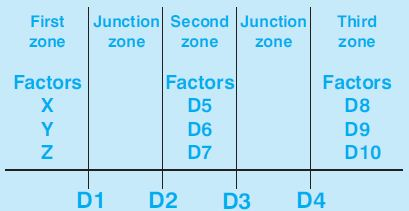# Selca G60 G61 Scaling factor

Selca G60 G61 Scaling factor

## G60 Cancels G61

G60 Cancels G61 (scaling factor)

`G60`

## G61 Scaling factor

G61 Scaling factor

### Programming

`G61 K...`
`G61 [X...] [Y...] [Z...] [F...] [Q...] [J...]`

### Parameters

ParameterDescription
Kscaling factor (multiplier) to be applied to all axes.
X,Y,Zscaling factor to be applied to each axis.
Fscaling factor to be applied to the feed rate.
Qscale factor to apply to the feed speed of axis movements perpendicular to the working plane ( for G17, for G18, for G19).
Jscale factor to apply to the rapid speed.

Enabled: until a G60 is programmed.

## G61 Programmable zone scaling

G61 Programmable zone scaling

### Programming

`G61 [X...] [Y...] [Z...] D0=... D1=... D2=... D3=... D4=... [D5=...] [D6=...] [D7=...] [D8=...] [D9=...] [D10=...]`### Parameters

ParameterDescription
Xscaling factor for X-axis first zone.
Yscaling factor for Y-axis first zone.
Zscaling factor for Z-axis first zone.
D0selection of the axis along which the 3 zones are defined:
D0=1 X-axis.
D0=2 Y-axis.
D0=3 Z-axis.
D1=end coordinate of the first zone.
D2=starting coordinate of the second zone (>D1).
D3=end coordinate of the second zone (>D2).
D4=starting coordinate of the third zone (>D3).
D5=scaling factor for X-axis second zone.
D6=scaling factor for Y-axis second zone.
D7=scaling factor for Z-axis second zone.
D8=scaling factor for X-axis third zone.
D9=scaling factor for Y-axis third zone.
D10=scaling factor for Z-axis third zone.

Enabled: until a G60 is programmed.